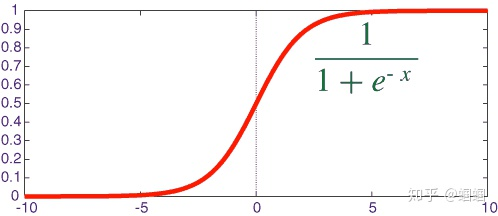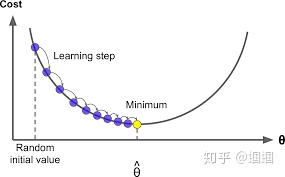# [深度学习笔记1]Logistic回归：最基础的神经网络

## 一、什么是logictic regression

Logistic Regression

x0w0 x1w1 ... x12287w12287 b=WTx bsigmoid function

y\' = σ(WTx b)

## 二、怎么学习W和b

L(y\',y) = -[y·log(y\') (1-y)·log(1-y\')]

• 当y=1时，L(y\',y)=-log(y\')，要使L最小，则y\'要最大，则y\'=1；
• 当y=0时，L(y\',y)=-log(1-y\')，要使L最小，则y\'要最小，则y\'=0.

X = (x(1)，x(2)，...，x(m) )

Y = (y(1)，y(2)，...，y(m) )

Y\' = (y\'(1)，y\'(2)，...，y\'(m) )

J(W,b) = 1/m·Σmi=1L(y\'(i),y(i)) 代表所有训练样本的平均损失。

Find W,b that minimize J(W,b)梯度下降法

w := w - α(dJ/dw)

## 总结一下：

• Logistic Regression模型：y\' = σ(WTx b)，记住使用的激活函数是sigmoid函数。
• 损失函数：L(y\',y) = -[y·log(y\') (1-y)·log(1-y\')]衡量预测值y\'与真实值y的差距，越小越好。
• 代价函数：损失均值，J(W,b) = 1/m·Σmi=1L(y\'(i),y(i))，是W和b的函数，学习的过程就是寻找W和b使得J(W,b)最小化的过程。求最小值的方法是用梯度下降法。
• 训练模型的步骤
1. 初始化W和b
2. 指定learning rate和迭代次数
3. 每次迭代，根据当前W和b计算对应的梯度（J对W，b的偏导数），然后更新W和b
4. 迭代结束，学得W和b，带入模型进行预测，分别测试在训练集合测试集上的准确率，从而评价模型

• 本文由 发表
• 转载请务必保留本文链接：https://www.plob.org/article/23935.html